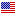1st Edition

# A Gentle Introduction to Scientific Computing

By Dan Stanescu, Long Lee Copyright 2022
ISBN 9780367206840
282 Pages 50 B/W Illustrations
Published May 2, 2022 by Chapman & HallFree Shipping (6-12 Business Days)
shipping options

USD \$110.00
ISBN 9780429262876
282 Pages 50 B/W Illustrations
Published May 1, 2022 by Chapman & Hall

Also available as eBook on:

Scientific Computation has established itself as a stand-alone area of knowledge at the borderline between computer science and applied mathematics. Nonetheless, its interdisciplinary character cannot be denied: its methodologies are increasingly used in a wide variety of branches of science and engineering.

A Gentle Introduction to Scientific Computing intends to serve a very broad audience of college students across a variety of disciplines. It aims to expose its readers to some of the basic tools and techniques used in computational science, with a view to helping them understand what happens "behind the scenes" when simple tools such as solving equations, plotting and interpolation are used.

To make the book as practical as possible, the authors explore their subject both from a theoretical, mathematical perspective and from an implementation-driven, programming perspective.

Features

• Middle-ground approach between theory and implementation.
• Suitable reading for a broad range of students in STEM disciplines. Could be used as the primary text for a first course in scientific computing.
• Introduces mathematics majors, without any prior computer science exposure, to numerical methods.
• All mathematical knowledge needed beyond Calculus (together with the most widely used Calculus notation and concepts) is introduced in the text to make it self-contained.

The erratum document for A Gentle Introduction to Scientific Computing can be accessed
here.

1. Introduction. 1.1. Scientific Computing. 1.2. MATLAB: what and why? 1.3. A Word of Caution. 1.4. Additional Resources. 2. Vectors and Matrices. 2.1. Unidimensional Arrays: Vectors. 2.2. Bidimensional Arrays: Matrices. 2.3. Matrix Operations. 2.4. Systems of Linear Equations. 2.5. Eigenvalues and Eigenvectors. 2.6. Operation Counts. 2.7. Exercises. 3. Basics of MATLAB. 3.1. Defining and Using Scalar Variables. 3.2. Saving and Reloading the Workspace. 3.3. Defining and Using Arrays. 3.4. Operations on Vectors and Matrices. 3.5. More on Plotting Functions of One Variable. 3.6. Loops and Logical Operators. 3.7. Working with indices and arrays. 3.8. Organizing Your Outputs. 3.9. Number representation. 3.10. Machine epsilon. 3.11. Exercises. 4. Solving Nonlinear Equations. 4.1. The Bisection Method for Root-Finding. 4.2. Convergence Criteria and Efficiency. 4.3. Scripts and Function Files. 4.4. The False Position Method. 4.5. The Newton—Raphson Method for Root-Finding. 4.6 Fixed Point Iteration. 4.7. MATLAB built-in functions. 4.8. Exercises. 5. Systems of Equations. 5.1. Linear Systems. 5.2. Newton’s Method for Nonlinear Systems. 5.3. MATLAB built-in functions. 5.4. Exercises. 6. Approximation of Functions. 6.1. A hypothetical example. 6.2. Global Polynomial Interpolation. 6.3. Spline Interpolation. 6.4. Approximation with Trigonometric Functions. 6.5. MATLAB built-in functions. 6.6. Exercises. 7. Numerical Differentiation. 7.1. Basic Derivative Formulae. 7.2. Derivative Formulae Using Taylor Series. 7.3. Derivative Formulae Using Interpolants. 7.4. Errors in Numerical Differentiation. 7.5. Richardson Extrapolation. 7.6. MATLAB built-in functions. 7.7. Exercises. 8. Numerical Optimization. 8.1. The need for optimization methods. 8.2. Line Search Methods. 8.3. Successive Parabolic Interpolation. 8.4. Optimization Using Derivatives. 8.5. Linear programming. 8.6. Constrained nonlinear optimization. 8.7. MATLAB built-in functions. 8.8. Exercises. 9. Numerical Quadrature. 9.1. Basic Quadrature Formulae. 9.2. Gauss Quadrature. 9.3. Extrapolation Methods: Romberg Quadrature. 9.4. Higher-Dimensional Integrals. 9.5. Monte Carlo Integration. 9.6. MATLAB built-in functions. 9.7. Exercises. 10. Numerical Solution of Differential Equations. 10.1. First-order Models. 10.2. Second-order Models. 10.3. Basic Numerical Methods. 10.4. Global error and the order of accuracy. 10.5. Consistency, Stability and Convergence. 10.6. Explicit vs. Implicit Methods. 10.7. Multistep Methods. 10.8. Higher-Order Initial Value Problems. 10.9. Boundary Value Problems. 10.10. MATLAB built-in functions. 10.11. Exercises. Appendix A. Calculus Refresher. A.1. Taylor Series. A.2. Riemann Integrals. A.3. Other Important Results. Appendix B. Introduction to Octave. B.1. The Problem of Choice. B.2. Octave Basics. B.3. Octave Code Examples. Appendix C. Introduction to Python. C.1. The problem of choice. C.2. Python Basics. C.3. Installing Python. C.4. Python Code Examples. Appendix D. Introduction to Julia. D.1. The problem of choice. D.2. Julia Basics. D.3. Julia Code Examples. Appendix E. Hints and Answers for Selected Exercises. Bibliography. Index.

### Biography

Dan Stanescu received his undergraduate degree in Aerospace Engineering from the Polytechnical Institute in Bucharest, Romania. He then pursued his graduate studies at McGill University and obtained his Ph.D. at Concordia University, both in Montreal, Canada. His initial fascination with modeling fluid flow led him to develop both frequency- and time-domain computational methods for the study of aircraft noise. His publications include work in aeroacoustics, spectral methods, stochastic processes and biomathematics. Upon joining the Mathematics Department at the University of Wyoming in 2003, he directed the Institute for Scientific Computing for six years and initiated an Interdisciplinary Computational Science Minor program, currently offered to interested students at both the undergraduate and graduate level. He has taught a wide range of courses in applied mathematics and has been developing and using the material included herein, during the last ten years, for a core class that is part of this Minor.

Long Lee is a Professor of Mathematics at the University of Wyoming in Laramie, USA. He received his Ph. D. in Applied Mathematics from the University of Washington in Seattle, USA. Before his Ph.D., he received a BS in Engineering and an MS in Geophysics.

His research interests are in the area of high-performance scientific computing and its applications. He has published papers in computational fluid dynamics, nonlinear waves, and image sciences. He expanded his research to infectious diseases and network modeling in recent years due to the COVID-19 pandemic.

His teaching interests are in the general area of scientific computing. In particular, he enjoys teaching numerical methods for engineering students. He has taught numerical methods, differential equations, and mathematical modeling, both undergraduate and graduate levels, at the University of Wyoming for more than fifteen years.

We offer free standard shipping on every order across the globe.